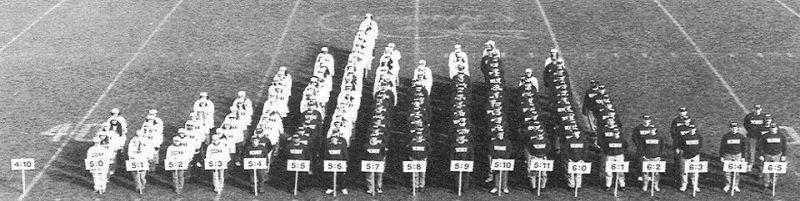by Kirill Dubovikov

# The t-distribution: a key statistical concept discovered by a beer breweryWhat does Guinness beer have to do with probability distributions? You’ll know by the end of this article.

In this post we will look at two probability distributions you will encounter almost each time you do data science, statistics, or machine learning.

### Gaussian distribution

Imagine that we are doing a research on the height of various people in a city. We go down the street and measure a bunch of random people. (Some of them thought this was quite strange and wanted to call the police, but come on, this is for the science!)

Now we decide that some Exploratory Data Analysis won’t hurt. But statistical software like R isn’t available at the moment, so we just make a histogram out of people.When you have no statistical software at hand…

What do we see here? Ahh, the famous bell curve. This is likely to be the most important probability distribution you will ever encounter. Thanks to the Central Limit Theorem, the Gaussian distribution is present in many real world phenomena. It’s so common that people just call it a normal distribution.

The Central Limit Theorem states that arithmetic mean of a sufficiently large number of independent random variables will be normally distributed. Those random variables can have any distribution initially. But when we measure something that is represented by their sum, we will eventually (as the number of samples tends to ) end up with normally distributed process.

The probability density function of Gaussian distribution is written below:

This formula may look a bit intimidating, but it’s convenient to work with mathematically. If you’re interested in how it can be derived, you can read how here. As you can see this distribution has two parameters:

• µ (mean)
• σ(standard deviation).

Mean µ controls the expected value (where the most values will go) of a normally distributed random variable. Variance σ² controls the spread or variety of possible values under the distribution.

The concept of a normal distribution has immense value in machine learning. A great variety of machine learning algorithms use it extensively:

• Linear models assume that errors are normally distributed
• Gaussian processes assume that all values of a function under the model are distributed normally
• Gaussian mixtures let you model complex distributions and build classifiers on top of mixture models
• Normal distribution comes up as one of the main components in Variational Autoencoders

Here is an interactive demo of the Gaussian distribution.

### A student’s t-distribution

What if we wanted to model our data with Gaussian distribution, but the variance σ² is was not known to us? This problem arises when the sample sizes are small and standard deviation (σ) can not be estimated accurately.

William Gosset tackled this problem while working at a Guinness brewery. He empirically found a formula for a t-distributed random variable.

First, suppose we have values x, …, xn which were sampled from some normal distribution N(µ, σ²).

We do not know the true variance, but we can estimate it by calculating sample mean and variance:

Then the random variable

will have a t-distribution with n-1 degrees of freedom, where n is the number of samples.

This formula may resemble transformation from Normal to Standard Normal (a shorthand for Normal distribution with zero mean and unit variance):

We don’t know the true population variance, so we have to substitute sample standard deviation estimate for the real one.

This distribution lies at the foundation of the scientific method, called the t-test. This was used at Guinness to measure the quality of their beer.

William Gosset published this result under a pseudonym Student. Guinness was afraid that its competitors would discover that the t-test was used to control the quality of their product.

Gosset’s discoveries were later formalized by famous statistician Ronald Fisher. Fisher is considered to be the author of the frequentist approach to statistics.

Now goes the fun part! You can play with t-distribution below:

As you can see t-distribution approaches standard normal when degrees of freedom are large. This happens because sample mean approaches true mean as a number of samples approaches infinity. The “fat” tails of t-distribution compensate for uncertainty when we are working with small samples.

An interested reader might ask, “So, what is the probability density function of the t-distribution? How can we derive it?” This turns out to be not that easy in terms of mathematics, but the central idea is easy to grasp.

Let’s suppose we are interested in getting the probability density function of normal variable X ~ N(0, σ). But without direct dependence on standard deviation σ.

Intuitively, to get rid of σ we must make some assumptions. Let’s treat σ as a random variable itself, and assume that it follows Gamma distribution (this is a very general distribution which has many uses in Bayesian statistics).

This way we may say that X is a mixture of two continuous probability distributions: Normal and Gamma. Then we integrate out σ and arrive at the probability density function formula for the t-distribution.

You can see more formal proofs here and here.

### Conclusion

Gaussian distributions and Student’s distributions are some of the most important continuous probability distributions in statistics and machine learning.

The t-distribution may be used as a placeholder for Gaussian when population variance is not known, or when the sample size is small. Both are closely related to each other in a strict and formal way.

Thanks for reading my article! I hope it helped you to learn something new or refresh existing knowledge.